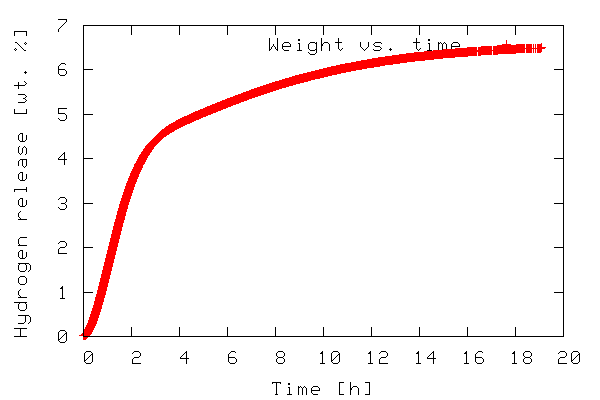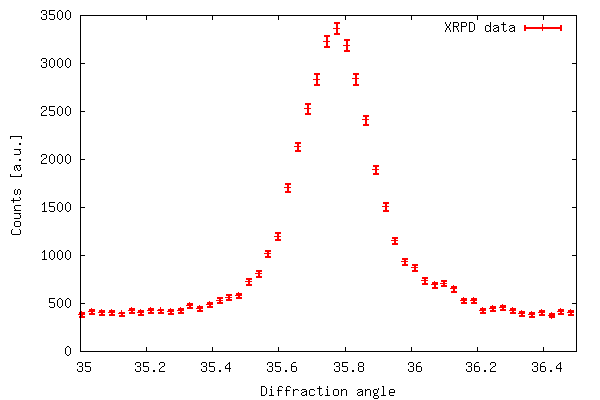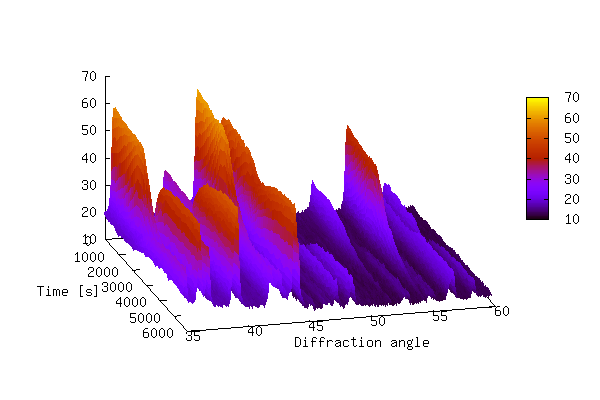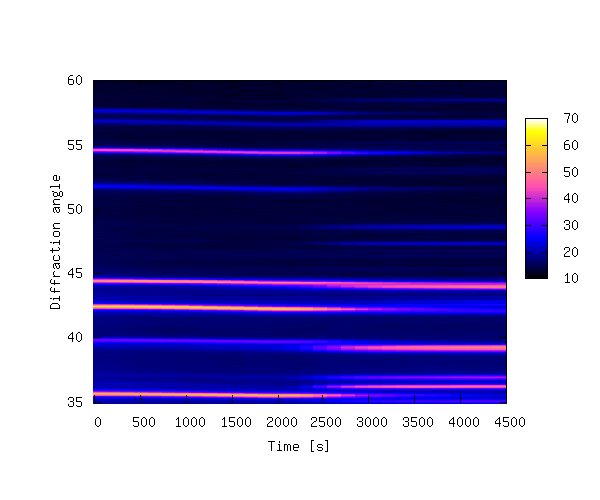...making Linux just a little more fun!

# Python for scientific use. Part I: Data Visualization

By Anders Andreasen

## Motivation and outline

A first step towards qualitative understanding and interpretation of scientific data is visualization of the data. Also, in order to reach a quantitative understanding, the data needs to be analyzed, e.g. by fitting a physical model to the data. The raw data may also require some initial processing in order to become useful, e.g. filtering, scaling, calibration etc.

Several open source programs for data analysis and visualization exist: gnuplot, grace, octave, R, and scigraphica. Each of these has its own pros and cons. However, it seems like you always end up using more than one program to cover all the different needs mentioned above, at least if you don't have the programming abilities to write your own custom programs using e.g., Fortran or C.

Recently, I came across Python and found it to be a very powerful tool. In this article, I would like to share my experience and illustrate that even with basic (or less) programming skills it is still possible to create some very useful applications for data analysis and visualization using this language. The article is centered around a few illustrative examples and covers the visualization part — data analysis will be covered in a future article.

## Python: a brief review

Python was originally created by Guido van Rossum and is an interpreted programming language (like e.g. Perl) with a clear and easy-to-read syntax. You can write stand-alone applications with Python, but one of it's strengths is its ability to act as glue between different kinds of programs.

The standard introduction to any programming language is the Hello world! program. In Python this is generated by first opening the Python interpreter by typing `python` on the command line. Your screen should look something like this:

```Python 2.3.4 (#1, Jan 21 2005, 11:24:24)
[GCC 3.3.3 20040412 (Gentoo Linux 3.3.3-r6, ssp-3.3.2-2, pie-8.7.6)] on linux2
>>>
```
Then the following code is typed:
```print "Hello world!"
```

Python code can also be stored in a file e.g. named `script.py`. By convention, files containing python code have a `*.py` extension. The script can be executed by typing `python script.py` on the command line. The program output will then be written to stdout and appear on the screen. If the following line is added to the top of the file:

```#! /usr/bin/python
```

(assuming that the python executable or a symlink to it exists) and giving the file executable mode with ```chmod u+x script.py``` the script can be executed by typing `./script.py` on the command line.

Python comes with many modules, either built-in or available for separate download and installation. In this article we will use SciPy, which is a very powerful library of modules for data visualization, manipulation and analysis. It adds functionality to Python making it comparable to e.g. octave and matlab.

## Plotting 2-D data

### Example 1: Plotting x,y data

The first example illustrates plotting a 2-D dataset. The data to be plotted is included in the file tgdata.dat and represents weight loss (in wt. %) as a function of time. The plotting routine is in the file tgdata.py and the python code is listed below. Line numbers have been added for readability.

```     1  from scipy import *
2
4  plotfile='tgdata.png'
5
6  gplt.plot(data[:,0],data[:,1],'title "Weight vs. time" with points')
7  gplt.xtitle('Time [h]')
8  gplt.ytitle('Hydrogen release [wt. %]')
9  gplt.grid("off")
10  gplt.output(plotfile,'png medium transparent picsize 600 400')
```

To run the code, download the tgdata.py.txt file, rename it to `tgdata.py`, and run it with ```python tgdata.py```. Besides Python, you also need SciPy and gnuplot installed. Gnuplot version 4.0 was used throughout this article. The output of the program is a plot to screen as shown below. The plot is also saved to disk as `tgdata.png` per line 4 above.In line 1, everything from the SciPy module is imported. In order to make use of the various functions of a module, the module needs to be imported by adding an `import module-name` line to the the python script. In this case it might have been sufficient to import only the `gplt` package and the `io.array_import` package. In line 3 the `io.array_import` package is used to import the data file `tgdata.dat` into the variable called `data` as an array with the independent variable stored in column 0 (note that array indices start with 0 as in C unlike Fortran/Octave/Matlab where it starts at 1) and the dependent variable in column 1. In line 4 a variable containing the file name (a string) to which the plot should be stored. In line 6-10 the `gplt` package is used as an interface to drive gnuplot. Line 6 tells gnuplot to use column 0 as x-values and column 1 as y-values. The notation `data[:,0]` means: use/print all rows in column 0. On the other hand `data[0,:]` refers to all columns in the first row.

The gnuplot png option `picsize` can be a little tricky. The example shown above works when Gnuplot is built with `libpng + zlib`. If you have Gnuplot built with `libgd` the required syntax becomes `size` and the specified width and height should be comma separated.

In order to plot a file with a different file name, we have to open the python source in a text editor and manually change the name of the file to be imported. We also need to change the name of the file copy if we do not want to overwrite the previous plot. This is a little tedious. We can easily add this functionality to our python script by allowing filenames to be passed as command line arguments. The modified script is called tgdata1.py and is shown below.

```     1  import sys, glob
2  from scipy import *
3
4  plotfile = 'plot.png'
5
6  if len(sys.argv) > 2:
7          plotfile = sys.argv
8  if len(sys.argv) > 1:
9          datafile = sys.argv
10  else:
11          print "No data file name given. Please enter"
12          datafile = raw_input("-> ")
13  if len(sys.argv) <= 2:
14          print "No output file specified using default (plot.png)"
15  if len(glob.glob(datafile))==0:
17          sys.exit()
18
20
21  gplt.plot(data[:,0],data[:,1],'title "Weight vs. time" with points')
22  gplt.xtitle('Time [h]')
23  gplt.ytitle('Hydrogen release [wt. %]')
24  gplt.grid("off")
25  gplt.output(plotfile,'png medium transparent picsize 600 400')
```

In the first line we have imported two new modules — `sys` and `glob` — in order to add the desired flexibility. In Python, `sys.argv` contains the command line arguments when execution starts. `sys.argv` contains the filename of the python script executed, `sys.argv` contains the first command line argument, `sys.argv` contains the second command line argument and so on. The `glob.glob()` function behaves as `ls` in *nix environments in that it supplies filename wildcarding. If no matches are found it returns the empty list (and thus has a len() of zero), otherwise it contains a list of matching filenames. The script can be executed with any desired number of command line arguments. If executed with two arguments e.g. `python tgdata1.py tgdata.dat tgdata1.png` the first argument is the name of the file containing the data to be plotted, and the second argument is the desired name of the file copy of the plot.

The script works as follows. A default file name for the hard copy of the plot is stored in the variable `plotfile` (line 4). Then some conditions about the number of given command line arguments are checked. First, if two or more command line arguments are given `plotfile` is overwritten with argument no. 2 (line 6-7.) Any arguments after the second are silently ignored. For 1 or more arguments given argument 1 is used as the data file name (line 8-9). If no command line arguments are passed, the user is prompted to input the name of the data file (line 10-12). In case of an invalid file name being used for the data file the script prints an error message and exits.

### Example 2: Plotting x,y data with error bars

So far we have shown that the `gplt` package is easy to interface with gnuplot and very effective. However, for scientific use it is often desirable to represent uncertainties for each data point. Although this is possible in gnuplot the `gplt` interface lacks this functionality. Instead we use the `popen` package included in the `os` module. With `popen` it is possible connect to the stdin (or stdout) of a program through a pipe.

The code below (also available in xrddata.py.txt, the data file is available as xrddata.dat) more or less shows how example 1 is reproduced using `popen` instead of the `gplt` package. The major difference is the fact that with `popen` it is not necessary to import the data to be plotted into Python - instead it is read directly by gnuplot.

```     1  import os
2
3  DATAFILE='xrddata.dat'
4  PLOTFILE='xrddata.png'
5  LOWER=35
6  UPPER=36.5
7
8  f=os.popen('gnuplot' ,'w')
9  print >>f, "set xrange [%f:%f]" % (LOWER,UPPER)
10  print >>f, "set xlabel 'Diffraction angle'; set ylabel 'Counts [a.u.]'"
11  print >>f, "plot '%s' using 1:2:(sqrt(\$2)) with errorbars title 'XRPD data' lw 3" % DATAFILE
12  print >>f, "set terminal png large transparent size 600,400; set out '%s'" % PLOTFILE
13  print >>f, "pause 2; replot"
14  f.flush()
```

The code of example 2 produces the output shown below. The error bar plot is created with ```plot 'filename' using 1:2:(sqrt(\$2)) with errorbars``` because in xrddata.dat the standard deviations are equal to the square root of the y-values. This is a special case and usually errors are given explicitly as a third data column in the data file. Thus, an error bar plot is created with `plot 'filename' using 1:2:3 with errorbars`.## Plotting 3D-data

### Example 3:

Now we look at how 3-D data can be represented by using a combination of Python/gnuplot. In order for gnuplot to represent 3-D data it requires that the data is either given by a mathematical expression or stored in a data file. The data file should have either a matrix-format where z-values are given as a matrix with x and y equal to the row and column number, respectively, corresponding to each z-value or a 3 column format where each row represents a data triple with x, y, and z given by the 1., 2., and 3. column respectively. See the gnuplot manual for further details.

The data to be represented in a 3-D fashion in this example is actually a collection of 2-D data files (like the one shown in example 2). Each data file corresponds to an experiment at a different time (with 150 s intervals between experiments) so we have two independent variables and one dependent variable: x(file number/time), y (diffraction angle),and z(counts) distributed across several files. This makes the 3-D data not suitable for plotting — yet.

The script 3ddata_1.py shown below finds all files with a given extension (*.x_y in this case) in the current working directory and creates a list containing their file names (line 5, `FILELIST`). In line 6 the number of data rows in each file is determined (`SIZEX`). This information, including the number of data files, is then used to construct an array (`DATAMATRIX`) with a size of `SIZEX` by `len(FILELIST)`. In lines 11-12 we cycle through the data files copying the second column in datafile number y is copied to column number y in `DATAMATRIX`. The array now holds all z-values. This array is only temporary, suitable for data processing before the actual data file for the 3-D plotting is written.

In line 14-22 the data file is written in the (x,y,z) format with x corresponding to time (found by multiplying the file number with the time step), y corresponding to diffraction angle as given by `TWOTHETA`, z corresponding to counts. In this case we only want to plot the data with diffraction angles between 35-60 (corresponding to data rows 1126-1968). Therefore, only this range is written to file in order to speed up both the process of writing to file and the plotting. In line 24-29 gnuplot is fed input using the `popen` package.

```     1  import os, glob
2  from scipy import *
3
4  EXT='*.x_y'
5  FILELIST=glob.glob(EXT)
7  DATAMATRIX = zeros((SIZEX,len(FILELIST)), Float)
9  TIMESTEP=150
10
11  for y in range(len(FILELIST)):
13
14  file = open("3ddata.dat", "w")
15
16  for y in range(len(FILELIST)):
17          for x in range(1126,1968):
18                  file.write(repr(TIMESTEP*y)+" "\
19                  +repr(TWOTHETA[x])+" "+repr(DATAMATRIX[x,y]))
20                  file.write("\n")
21          file.write("\n")
22  file.close()
23
24  f=os.popen('gnuplot' ,'w')
25  print >>f, "set ticslevel 0.0 ;set xlabel 'Time [s]'; set ylabel 'Diffraction angle'"
26  print >>f, "set pm3d; unset surface; set view 60,75; splot '3ddata.dat' notitle"
27  print >>f, "set terminal png large transparent size 600,400; set out '3ddata_1.png'"
28  print >>f, "replot"
29  f.flush()
```

If you wanted to write a 3-D data file in the matrix-format, lines 14 through 22 can be replaced with the following code.

```file = open("3ddata_matrix.dat", "w")
for x in range(SIZEX):
for y in range(len(FILELIST)):
file.write(repr(DATAMATRIX[x,y])+" ")
file.write("\n")
file.close()
```

The 3-D plot produced by the above script is shown below.The plot above shows that it can be difficult to produce a 3-D plot of non-monotonic data that shows all of the details of the data — some of the smaller peaks are hidden behind larger peaks. It is also difficult to see changes in peak positions as a function of time. In order to bring out these details it is sometimes better to create a 2-D contour plot by projecting the z-values down into the x,y plane. This is achieved by replacing lines 24-29 of 3ddata_1.py with the code below (3ddata_2.py).

```f=os.popen('gnuplot' ,'w')
print >>f, "set pm3d map; set palette rgbformulae 30,31,32; set xrange[0:4500]"
print >>f, "set xlabel 'Time [s]'; set ylabel 'Diffraction angle'"
print >>f, "splot '3ddata.dat' notitle"
print >>f, "set terminal png large transparent size 600,500; set out '3ddata.png'"
print >>f, "replot"
f.flush()
```

The contour plot is shown below.The 3-D example plots were made with 39 data files, each containing 4096 data rows. The data files are available at this offsite link, and can be unpacked with `tar xvfz 3ddata.tar.gz`.

## Summary

In this article a few examples have been given in order to illustrate that Python is indeed a powerful tool for visualization of scientific data by combining the plotting power of gnuplot with the power of a real programming language. It should be noted that all the examples given here could probably have been solved with a combination of e.g. `bash`, `gawk` and gnuplot. It appears to me that Python is much simpler and the resulting scripts are more transparent and easy to read and maintain. If heavy data processing is required the bash/gawk/gnuplot might also need the added functionality of e.g. octave. With Python this functionality is in SciPy.

Manuals, Tutorials, Books etc:

1. Guido van Rossum, Python tutorial, http://docs.python.org/tut/tut.html
2. Guido van Rossum, Python library reference, http://docs.python.org/lib/lib.html
3. Mark Pilgrim, Dive into Python, http://diveintopython.org/toc/index.html
4. Thomas Williams & Colin Kelley, Gnuplot - An Interactive Plotting Program, http://www.gnuplot.info/docs/gnuplot.html
5. Travis E. Oliphant, SciPy tutorial, http://www.scipy.org/documentation/tutorial.pdf
6. David Ascher, Paul F. Dubois, Konrad Hinsen, Jim Hugunin and Travis Oliphant, Numerical Python, http://numeric.scipy.org/numpydoc/numdoc.htm

## Other graphical libraries for Python

Gnuplot version 4.0 has been used in the examples throughout this article. Python also works with other plotting engines/libraries:

1. Grace, see http://www.idyll.org/~n8gray/code/
2. PGPLOT, see http://efault.net/npat/hacks/ppgplot/
3. PLplot, see http://www.plplot.org/
4. matplotlib, see http://matplotlib.sourceforge.net/ (library specifically made for Python)Anders has been using Linux for about 6 years. He started out with RH 6.2, moved on to RH 7.0, 7.1, 8.0, Knoppix, has been experimenting a little with Mandrake, Slackware, and FreeBSD, and is now running Gentoo on his workstation (no dual boot :-) at work and Debian Sarge on his laptop at home. Anders has (a little) programming experience in C, Pascal, Bash, HTML, LaTeX, Python, and Matlab/Octave.

Anders has a Masters degree in Chemical Engineering and is currently employed as a Ph.D. student at the Materials Research Department, Risö National Laborary in Denmark. Anders is also the webmaster of Hydrogen storage at Risö.

Copyright © 2005, Anders Andreasen. Released under the Open Publication license unless otherwise noted in the body of the article. Linux Gazette is not produced, sponsored, or endorsed by its prior host, SSC, Inc.

Published in Issue 114 of Linux Gazette, May 2005